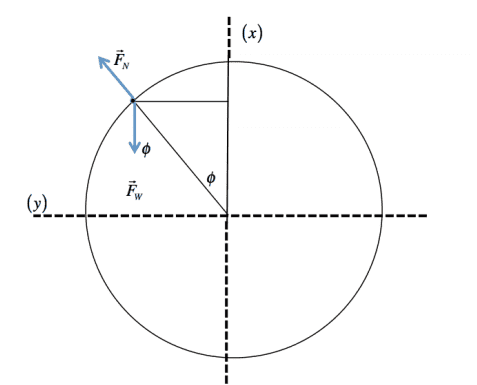# Kinematics in Cylindrical Coordinates

• Zinggy

## Homework Statement

A small bead of mass m slides on a frictionless cylinder of radius R which lies with its cylindrical axis horizontal. At t = 0 , when the bead is at (R,0), vz = 0 and the bead has an initial angular momentum Lo < mR sqrt(Rg) about the axis of the cylinder where g is the acceleration due to gravity. The bead slides from (R,0) down the curved surface of the cylinder and eventually loses contact with that surface.

Find r(double dot) in Cylindrical Coordinates.
2. Homework Equations

Position Vector of r = ˆ iRcosφ + ˆ jRsinφ = ρˆR

## The Attempt at a Solution

I know r(double dot) is the same as d2r/dt2 so;
r = ˆ iRcosφ + ˆ jRsinφ = ρˆR
r(dot) =- iRsinφ+jRcosφ -unless R changes with time, which I don't think it does.
then
r(double dot) = iR-cosφ - jRsinφ
I think I'm missing something. Do I need to include the angular momentum somewhere?

## Homework Statement

A small bead of mass m slides on a frictionless cylinder of radius R which lies with its cylindrical axis horizontal. At t = 0 , when the bead is at (R,0), vz = 0 and the bead has an initial angular momentum Lo < mR sqrt(Rg) about the axis of the cylinder where g is the acceleration due to gravity. The bead slides from (R,0) down the curved surface of the cylinder and eventually loses contact with that surface.

Find r(double dot) in Cylindrical Coordinates.
2. Homework Equations

Position Vector of r = ˆ iRcosφ + ˆ jRsinφ = ρˆR

## The Attempt at a Solution

I know r(double dot) is the same as d2r/dt2 so;
r = ˆ iRcosφ + ˆ jRsinφ = ρˆR
r(dot) =- iRsinφ+jRcosφ -unless R changes with time, which I don't think it does.
then
r(double dot) = iR-cosφ - jRsinφ
I think I'm missing something. Do I need to include the angular momentum somewhere?
You are missing the force of gravity in your equations.

r(dot) =- iRsinφ+jRcosφ -unless R changes with time, which I don't think it does.
Your are also missing the chain rule when you take derivatives with respect to time.
Is the bead on the inside or the outside of the cylinder? Specifically, is point (R,0) in Cartesian coordinates?

You are missing the force of gravity in your equations.

Your are also missing the chain rule when you take derivatives with respect to time.
Is the bead on the inside or the outside of the cylinder? Specifically, is point (R,0) in Cartesian coordinates?

This is the diagram that goes with the question.The bead is sliding along the outside of the cylinder. When I'm missing gravity, does it go in the first derivative? The position vector is given without gravity present.

#### Attachments

This is the diagram that goes with the question.
View attachment 238105
The bead is sliding along the outside of the cylinder. When I'm missing gravity, does it go in the first derivative? The position vector is given without gravity present.